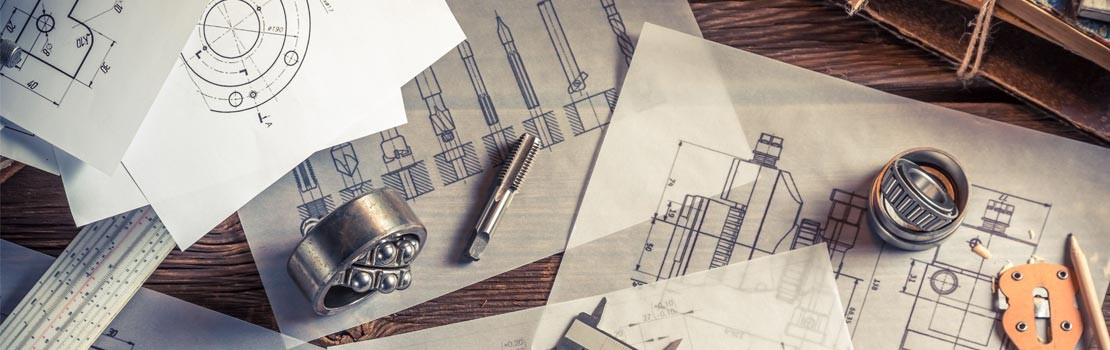Select Page
Home » Blog » Blog » How to Measure your Heat Load## Formula for Specific Heat

The first step in selecting the proper product for your cooling system application is to determine the heat load, or the amount of heat generated by your system. This article explains how to establish heat load for any liquid cooling application.

A quick and dirty method of estimating heat load is to assume that all electrical energy entering a process is converted to heat. From the 1st Law of Thermodynamics we know that the amount of energy exiting a system can never be greater than the amount of energy entering a system. The heat load can be conservatively estimated to be equal to the amount of electricity consumed if electricity is the only form of energy entering a system.

To determine heat load more accurately, the equation Q = m x Cp x ΔT can be used where:

• Q = heat load (BTU/hr)
• m = mass flow rate (lb/hr)
• Cp = specific heat (BTU/lb °F)
• ΔT = change in temperature (°F)

## Test Setup for Calculating Heat Load

In order to determine Q using the above equation, it is necessary to obtain the values m and ΔT experimentally. To measure these values you will need the following equipment:

 Quantity Equipment Recommended Accuracy 2 Type “T” Thermocouple ± 0.2°F 1 Turbine Flow Meter; ± 1% of Reading

The thermocouples and flow meter can be used to measure fluid temperature change and flow rate of the cooling fluid when your system is at peak load operation (see Figure 1). Using the fluid’s specific heat (properties of commonly used fluids can be found in the Thermal Reference Guide in our Technical Library) and the above equation, heat load can be computed.

The accuracy of the thermocouples and flow meter is particularly important, as a small decrease in accuracy can cause a significant error percentage. For example: if the temperature rise is 10°F and the thermocouples are accurate to ±0.5°F, the temperature rise measurement could be off by as much as 1°F, or 10%. This dictates that the entire heat load calculation cannot be more accurate than the same ±10%. If the temperature rise is less than 10°F, the ±0.5°F becomes an even higher error percentage. When the temperature error is given in °F or °C, the error percentage can be calculated by multiplying the thermocouple accuracy by two, then dividing by the change in temperature, and multiplying by one hundred.

Calibrating the two thermocouples prior to recording measurements is recommended. If this is not possible, the accuracy of one may be compared to the other. To do this, run a fluid stream through the thermocouples with no heat load. If the temperatures are the same, the exact temperature rise at peak operation can be used. Otherwise, the temperature difference of the two thermocouples under no heat load should be taken into account when conducting measurements at peak load operation. To do this subtract the temperature difference with no heat load from the temperature difference with heat load applied. For example: if the two thermocouples read 20.0 and 20.5°C when under no heat load, and 25.0 and 30.5°C with heat load applied, the change in temperature should be calculated to be (30.5-25.0)-(20.5-20.0), or 5.0°C.

If a flow meter is not available, a graduated container and a timer can be used to determine the fluid flow rate by measuring the amount of fluid that has passed through the system and dividing by the amount of time that has elapsed. A constant flow rate is essential when measuring the flow in this manner. The density of the fluid should be used to convert the volumetric flow rate to mass flow rate.

These methods of determining heat load are generic to any liquid cooling application and can be used when sizing a recirculating chiller, cold plate or heat exchanger.